# Texas Go Math Kindergarten Lesson 4.5 Answer Key Model, Count and Write 8

Refer to our Texas Go Math Kindergarten Answer Key Pdf to score good marks in the exams. Test yourself by practicing the problems from Texas Go Math Kindergarten Lesson 4.5 Answer Key Model, Count and Write 8.

## Texas Go Math Kindergarten Lesson 4.5 Answer Key Model, Count and Write 8

Explore

DIRECTIONS: Model 7 objects. Show one more object. How many objects are there now? Tell a friend how you know. Draw the objects.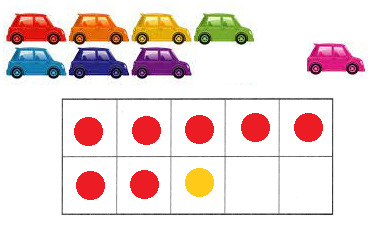Explanation:
I drew 7 cars and 1 more car.There are 8 cars in all.I drew 8 counters to show the number of cars.

Share and Show

DIRECTIONS: 1. Place counters as shown. Count and tell how many counters. Trace and write the numbers. Trace the word. 2. Place counters in the ten frame to model eight. Draw the counters. Tell a friend what you know about the number 8.

Question 1.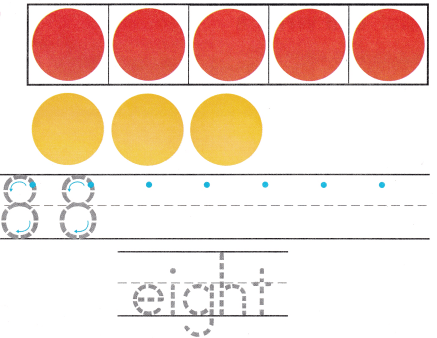Explanation:
I traced and practiced writing the number 8.
I traced the word eight.

Question 2.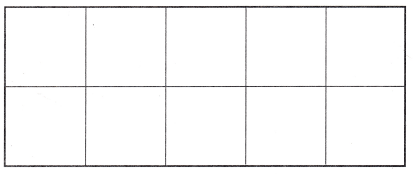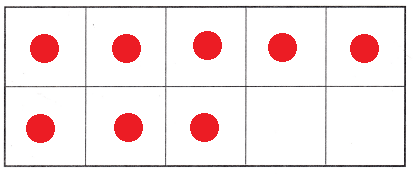Explanation:
I drew 8 counters to model the number 8.

DIRECTIONS: 3. Write the number 8. Place two-color counters in the ten frame to model the different ways to make 8. Write to show some pairs of numbers that make 8.

Question 3.Explanation:
I placed two-color counters 5 red and 3 yellow counters in the ten frame to model the different ways to make 8 and wrote to show some pairs of numbers that make 8, they are 5 red and 3 yellow, 4 and 4, 3 and 5 and 2 and 6.

HOME ACTIVITY • Ask your child to show a set of seven objects. Have him or her show one more object and tell how many.

DIRECTIONS: 4. Count the balls in each set. Which sets show eight balls? Circle those sets. 5. Choose the correct answer. How many parrots are there?

Problem Solving

Question 4.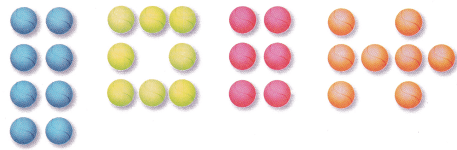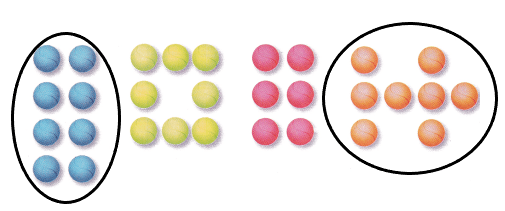Explanation:
I drew circle around the groups of balls that are 8 in number.

Question 5.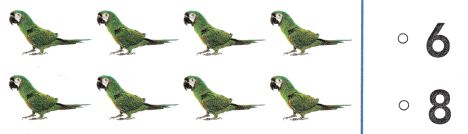Explanation:
I counted the number of birds.They are 8 in number.So, i marked the number 8.

### Texas Go Math Kindergarten Lesson 4.5 Homework and Practice Answer Key

DIRECTIONS: 1. Draw some red and yellow counters to make 8. Write to show the number of red and yellow counters. 2. Draw some red and yellow counters to make 8 another way. Write to show the number of red and yellow counters.

Question 1.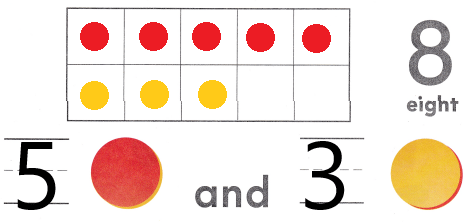Explanation:
I drew 5 red and 3 yellow counters to make 8 and wrote the numbers 5 and 3 to show the number of red and yellow counters.

Question 2.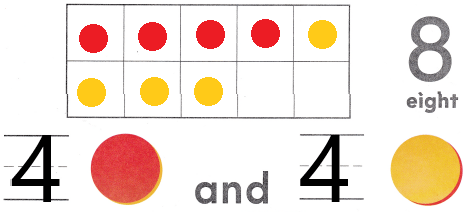Explanation:
I drew 4 red and 4 yellow counters to make 8 and wrote the numbers 4 and 4 to show the number of red and yellow counters.

DIRECTIONS: Choose the correct answer. 3. What number does the model slow? 4. How many puppies are there? 5. How many kittens are there?

Lesson Check

Question 3.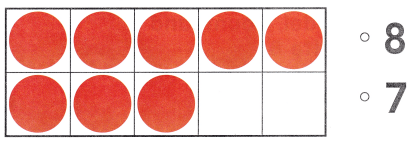Explanation:
There are 89 counters in the ten-frame.So, i marked the number 8.

Question 4.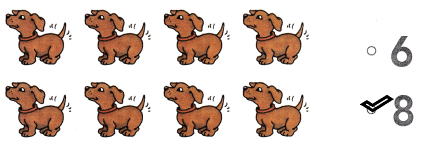Explanation:
I counted the number of puppies.They are 8 in number.So, i marked the number 8.

Question 5.